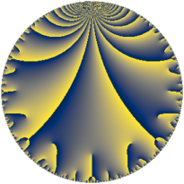# Properties

 Label 115.7.c.cLevel $115$ Weight $7$ Character orbit 115.c Analytic conductor $26.456$ Analytic rank $0$ Dimension $68$ CM no Inner twists $4$

# Related objects

Show commands: Magma / PariGP / SageMath

## Newspace parameters

comment: Compute space of new eigenforms

[N,k,chi] = [115,7,Mod(114,115)]

mf = mfinit([N,k,chi],0)

lf = mfeigenbasis(mf)

from sage.modular.dirichlet import DirichletCharacter

H = DirichletGroup(115, base_ring=CyclotomicField(2))

chi = DirichletCharacter(H, H._module([1, 1]))

N = Newforms(chi, 7, names="a")

//Please install CHIMP (https://github.com/edgarcosta/CHIMP) if you want to run this code

chi := DirichletCharacter("115.114");

S:= CuspForms(chi, 7);

N := Newforms(S);

 Level: $$N$$ $$=$$ $$115 = 5 \cdot 23$$ Weight: $$k$$ $$=$$ $$7$$ Character orbit: $$[\chi]$$ $$=$$ 115.c (of order $$2$$, degree $$1$$, minimal)

## Newform invariants

comment: select newform

sage: f = N # Warning: the index may be different

gp: f = lf \\ Warning: the index may be different

 Self dual: no Analytic conductor: $$26.4562196163$$ Analytic rank: $$0$$ Dimension: $$68$$ Twist minimal: yes Sato-Tate group: $\mathrm{SU}(2)[C_{2}]$

## $q$-expansion

The dimension is sufficiently large that we do not compute an algebraic $$q$$-expansion, but we have computed the trace expansion.

 $$\operatorname{Tr}(f)(q) =$$ $$68 q - 2440 q^{4} + 352 q^{6} - 16908 q^{9}+O(q^{10})$$ 68 * q - 2440 * q^4 + 352 * q^6 - 16908 * q^9 $$\operatorname{Tr}(f)(q) =$$ $$68 q - 2440 q^{4} + 352 q^{6} - 16908 q^{9} + 66968 q^{16} - 30916 q^{24} + 32588 q^{25} - 22072 q^{26} + 103360 q^{29} - 17256 q^{31} - 358168 q^{35} + 451984 q^{36} + 192432 q^{39} - 183552 q^{41} - 397956 q^{46} + 806756 q^{49} - 749960 q^{50} - 1638436 q^{54} - 1752 q^{55} - 505552 q^{59} - 4095100 q^{64} + 1354876 q^{69} + 1196604 q^{70} + 493688 q^{71} + 3178568 q^{75} + 2473820 q^{81} + 3306336 q^{85} - 3770196 q^{94} + 896144 q^{95} + 16928136 q^{96}+O(q^{100})$$ 68 * q - 2440 * q^4 + 352 * q^6 - 16908 * q^9 + 66968 * q^16 - 30916 * q^24 + 32588 * q^25 - 22072 * q^26 + 103360 * q^29 - 17256 * q^31 - 358168 * q^35 + 451984 * q^36 + 192432 * q^39 - 183552 * q^41 - 397956 * q^46 + 806756 * q^49 - 749960 * q^50 - 1638436 * q^54 - 1752 * q^55 - 505552 * q^59 - 4095100 * q^64 + 1354876 * q^69 + 1196604 * q^70 + 493688 * q^71 + 3178568 * q^75 + 2473820 * q^81 + 3306336 * q^85 - 3770196 * q^94 + 896144 * q^95 + 16928136 * q^96

## Embeddings

For each embedding $$\iota_m$$ of the coefficient field, the values $$\iota_m(a_n)$$ are shown below.

For more information on an embedded modular form you can click on its label.

comment: embeddings in the coefficient field

gp: mfembed(f)

Label $$a_{2}$$ $$a_{3}$$ $$a_{4}$$ $$a_{5}$$ $$a_{6}$$ $$a_{7}$$ $$a_{8}$$ $$a_{9}$$ $$a_{10}$$
114.1 4.94808i 27.5624i 39.5165 31.3018 121.017i 136.381 −630.980 512.208i −30.6878 −598.804 154.884i
114.2 4.94808i 27.5624i 39.5165 31.3018 + 121.017i 136.381 −630.980 512.208i −30.6878 −598.804 + 154.884i
114.3 15.5178i 42.9237i −176.801 113.739 51.8501i 666.080 −624.197 1750.41i −1113.45 −804.597 1764.97i
114.4 15.5178i 42.9237i −176.801 113.739 + 51.8501i 666.080 −624.197 1750.41i −1113.45 −804.597 + 1764.97i
114.5 11.1333i 17.3572i −59.9514 −121.447 + 29.5914i −193.244 504.412 45.0744i 427.728 329.451 + 1352.11i
114.6 11.1333i 17.3572i −59.9514 −121.447 29.5914i −193.244 504.412 45.0744i 427.728 329.451 1352.11i
114.7 10.4494i 30.8468i −45.1897 41.2139 + 118.010i −322.330 505.735 196.556i −222.524 1233.13 430.660i
114.8 10.4494i 30.8468i −45.1897 41.2139 118.010i −322.330 505.735 196.556i −222.524 1233.13 + 430.660i
114.9 14.4471i 3.70784i −144.720 95.5216 80.6265i −53.5677 454.114 1166.17i 715.252 −1164.82 1380.01i
114.10 14.4471i 3.70784i −144.720 95.5216 + 80.6265i −53.5677 454.114 1166.17i 715.252 −1164.82 + 1380.01i
114.11 8.96761i 45.1542i −16.4180 −49.9957 + 114.566i −404.925 −395.172 426.697i −1309.90 1027.39 + 448.342i
114.12 8.96761i 45.1542i −16.4180 −49.9957 114.566i −404.925 −395.172 426.697i −1309.90 1027.39 448.342i
114.13 4.35786i 24.6249i 45.0090 108.536 + 62.0072i 107.312 −356.743 475.046i 122.613 270.219 472.986i
114.14 4.35786i 24.6249i 45.0090 108.536 62.0072i 107.312 −356.743 475.046i 122.613 270.219 + 472.986i
114.15 11.1327i 32.3873i −59.9364 122.088 + 26.8239i 360.557 301.337 45.2393i −319.938 298.622 1359.17i
114.16 11.1327i 32.3873i −59.9364 122.088 26.8239i 360.557 301.337 45.2393i −319.938 298.622 + 1359.17i
114.17 0.0499926i 38.9668i 63.9975 95.0255 + 81.2106i 1.94805 −273.692 6.39893i −789.409 4.05993 4.75057i
114.18 0.0499926i 38.9668i 63.9975 95.0255 81.2106i 1.94805 −273.692 6.39893i −789.409 4.05993 + 4.75057i
114.19 7.58308i 53.1699i 6.49696 −65.6908 + 106.347i 403.191 −232.063 534.584i −2098.04 806.439 + 498.138i
114.20 7.58308i 53.1699i 6.49696 −65.6908 106.347i 403.191 −232.063 534.584i −2098.04 806.439 498.138i
See all 68 embeddings
 $$n$$: e.g. 2-40 or 990-1000 Embeddings: e.g. 1-3 or 114.68 Significant digits: Format: Complex embeddings Normalized embeddings Satake parameters Satake angles

## Inner twists

Char Parity Ord Mult Type
1.a even 1 1 trivial
5.b even 2 1 inner
23.b odd 2 1 inner
115.c odd 2 1 inner

## Twists

By twisting character orbit
Char Parity Ord Mult Type Twist Min Dim
1.a even 1 1 trivial 115.7.c.c 68
5.b even 2 1 inner 115.7.c.c 68
23.b odd 2 1 inner 115.7.c.c 68
115.c odd 2 1 inner 115.7.c.c 68

By twisted newform orbit
Twist Min Dim Char Parity Ord Mult Type
115.7.c.c 68 1.a even 1 1 trivial
115.7.c.c 68 5.b even 2 1 inner
115.7.c.c 68 23.b odd 2 1 inner
115.7.c.c 68 115.c odd 2 1 inner

## Hecke kernels

This newform subspace can be constructed as the intersection of the kernels of the following linear operators acting on $$S_{7}^{\mathrm{new}}(115, [\chi])$$:

 $$T_{2}^{34} + 1698 T_{2}^{32} + 1305039 T_{2}^{30} + 601112923 T_{2}^{28} + 185197947420 T_{2}^{26} + 40339513938099 T_{2}^{24} + \cdots + 92\!\cdots\!00$$ T2^34 + 1698*T2^32 + 1305039*T2^30 + 601112923*T2^28 + 185197947420*T2^26 + 40339513938099*T2^24 + 6402713046671032*T2^22 + 752220422766183060*T2^20 + 65786482989133982640*T2^18 + 4272451831158310641872*T2^16 + 203967217221957232494336*T2^14 + 7026156853296649578511296*T2^12 + 169642884121489664493208320*T2^10 + 2747988838469983980568117248*T2^8 + 27898894162686000118743760896*T2^6 + 157394554323651741722087522304*T2^4 + 370634158193196860144055484416*T2^2 + 925327957659603472849305600 $$T_{7}^{34} - 2201722 T_{7}^{32} + 2149633757328 T_{7}^{30} + \cdots - 29\!\cdots\!00$$ T7^34 - 2201722*T7^32 + 2149633757328*T7^30 - 1232490036737775930*T7^28 + 463440934025480403205548*T7^26 - 120938604458705133545860054680*T7^24 + 22615672160281067206353739556803625*T7^22 - 3086669814407091578252988859691276749250*T7^20 + 310357008889143305384588111616426676798905000*T7^18 - 23044807925649701593507808782624965225077786950000*T7^16 + 1258815528163973799864833992140205367525686053594250000*T7^14 - 50066638833908919382725656985511700295077983659282172500000*T7^12 + 1424302379526093608413091359680921764570397748538645251200000000*T7^10 - 28206302360033359012576474008522605653158664084521943832895000000000*T7^8 + 373669982880195820314026915503753689064130562467855859575796750000000000*T7^6 - 3116059493149372537417487896137324452401594686534888282811194712500000000000*T7^4 + 14646069288754117736200710464251190072962755445968358644071060460000000000000000*T7^2 - 29388755874626735497450336034789963884252209751161694978114229160000000000000000000